# Determine the number

Determine the number x that
$x+8$
is
$\frac{7}{5}$
from one half from
$7x-1$
.

x =  2.23

### Step-by-step explanation: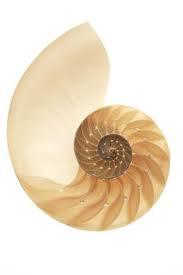Did you find an error or inaccuracy? Feel free to write us. Thank you!Tips to related online calculators
Need help to calculate sum, simplify or multiply fractions? Try our fraction calculator.
Do you have a linear equation or system of equations and looking for its solution? Or do you have a quadratic equation?

## Related math problems and questions:

• Two fraction equation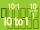Find d value of q in the equation 30/16=q/48.
• Number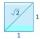Is number 5.146852 irrational?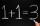Number 839 divide into the two addends that the first was 17 greater than 60% of the second. Determine these addends.
• Unknown number 716% of the unknown number is by 21 less than unknown number itself. Determine the natural unknown number.
• Strange x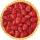For what x is true x=12x?
• Unknown numberFind an unknown number of which 1/5 is 40 greater than one-tenth of that number.
• Unknown numberIf I reduced the sum of the numbers 70 and the unknown number three times, I would get 100. what is the unknown number?
• A numberA number increased by 7.9 is 8.3
• Two thirdsTwo thirds of the unknown number is 8/7. What is 7/3 of this number?
• UN 1If we add to an unknown number his quarter, we get 210. Identify unknown number.
• Find the 11Find the quotient of 229.12 and 12.32
• Seven-segmet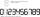Lenka is amused that he punched a calculator (seven-segment display) numbers and used only digits 2 to 9. Some numbers have the property that their image in the axial or central symmetry was again give some number. Determine the maximum number of three-di
• Two numbers 11The sum of two rational numbers is (-2). If one of them is 3/5, find the other.
• TV commercials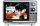For the typical one-hour prime-time television slot, the number of minutes of commercials is 3/8 of the actual program's minutes. Determine how many minutes of the program are shown in that one hour.
• Two numbersThe sum of two numbers is 1. Identify this two numbers if you know that the half of first is equal to the third of second number.
• Determine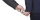Determine the salary of a part-time worker if the employer deducted (taxes etc) CZK 840 from him, which was 6/50 of his salary.
• FractionsThree-quarters of an unknown number is 4/5. What is 5/6 of this unknown number?